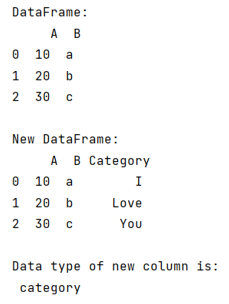# Python - Create a categorical type of column in pandas dataframe

Given a Pandas DataFrame, we have to create a categorical type of column. By Pranit Sharma Last updated : September 26, 2023

Pandas is a special tool that allows us to perform complex manipulations of data effectively and efficiently. Inside pandas, we mostly deal with a dataset in the form of DataFrame. DataFrames are 2-dimensional data structures in pandas. DataFrames consist of rows, columns, and data.

## Categorical data

Categorical data is a type of data that has some certain category or characteristic, the value of categorical data is not a single value, rather it consists of classified values, for example, an email can be considered as spam or not spam, if we consider 1 as spam and 0 as not spam, we have a classified data in the form of 0 or 1, this is called categorical data.

## Problem statement

Given a Pandas DataFrame, we have to create a categorical type of column.

## Creating a categorical type of column in pandas dataframe

Pandas allow us to create a categorical type column in pandas DataFrame by using pd.category() method, inside which we pass a list of values that we want in the column. For this purpose, we will first create a DataFrame, then we will add an extra column and assign this category types of values into this column.

Let us understand with the help of an example,

## Python program to create a categorical type of column

```# Importing pandas library
import pandas as pd

# Creating a dictionary
d = {
'A':[10,20,30],
'B':['a','b','c']
}

# Creating a dataframe
df = pd.DataFrame(d)

# Display Dataframe
print("DataFrame:\n",df,"\n")

df['Category'] = pd.Categorical(['I', 'Love', 'You'])

# Display modified DataFrame and dtype of new column
print("New DataFrame:\n",df,"\n")
print("Data type of new column is:\n",df['Category'].dtype)
```

### Output

The output of the above program is: